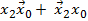# When the output Y in the circuit below is “1”, it implies that data has

1.   Changed from “0” to “1”

2.  Changed from “1” to “0”

3.  Changed in either direction

4.   Not changed

4

Changed from “0” to “1”

Explanation :
No Explanation available for this question

# Which of the following condition, clocked J-K flip-flop can be used as a divide-by-2 circuit when input signal is applied at clock input

1.  J=K=1 and Flip-Flop has active High Inputs

2.  J=K=0 and Flip-Flop has active Low Inputs

3.  J=K=1 and Flip-Flop has active Low Inputs

4.  J=K=1 and Flip-Flop Should be negative edge triggerd

4

J=K=1 and Flip-Flop has active High Inputs

Explanation :
No Explanation available for this question

# The counter circuit shown below will reset at

1.  8th input pulse

2.  10 th input pulse

3.  12 th input pulse

4.  16 th input pulse

4

10 th input pulse

Explanation :
No Explanation available for this question

# Consider the given circuit. In this circuit, the race around

1.   Does not occur

2.  Occurs when CLK=0

3.  Occurs when CLK=1 and A=B=1

4.  Occurs when CLK=1 and A=B=0

4

Does not occur

Explanation :
No Explanation available for this question

# The circuit shown below is

1.  Mod-4-counter

2.  Mod-5-counter

3.  Mod 6 counter

4.  Mod 7 counter

4

Mod-4-counter

Explanation :
No Explanation available for this question

# The Instruction DAA

1.  Converts binary to BCD

2.  Converts BCD to binary

3.  Decrements accumulator

4.  Add contents of accumulator to accumulator

4

Converts binary to BCD

Explanation :
No Explanation available for this question

# Read the inaction given below Assume clock period of 0.5 µs The above program execution will display

1.  8CH at port 1

2.  00H at port 1

3.  FFH at port 1

4.  FFH to 00H at port 1

4

FFH to 00H at port 1

Explanation :
No Explanation available for this question

# When a sun routine is called, then address of the instruction following the CAL instruction is stored in/on the

1.  Stack pointer

2.  Accumulator

3.  Program counter

4.  Stack

4

Program counter

Explanation :
No Explanation available for this question

# f1(x2, x1, x0) will be

1.

2.

3.  x2⊕ x0

4.  x2 x0

4Explanation :
No Explanation available for this question

# The digital block in the figure is realized using two positive edge triggered D flip-flops. Assume that for t

1.

2.

3.

4.

4